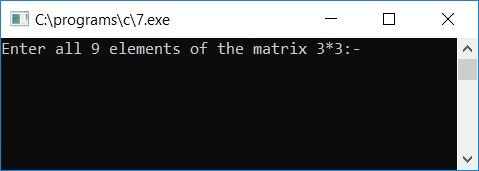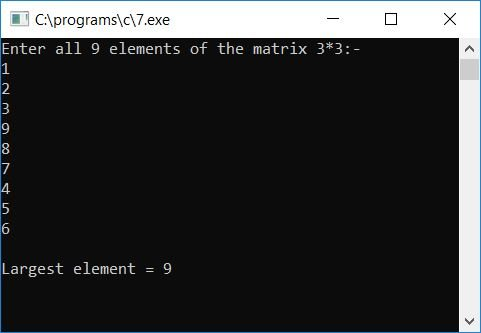# C Program to Find Largest Element in a Matrix

In this article, we will learn how to create a program in C that will find and print the largest element from a matrix. This program will ask the user to enter any 3-by-3 matrix and then find out the largest element from that given 3-by-3 matrix (by the user at run-time).

```#include<stdio.h>
#include<conio.h>
int main()
{
int mat, i, j, max;
printf("Enter any 3*3 matrix: ");
for(i=0; i<3; i++)
{
for(j=0; j<3; j++)
scanf("%d", &mat[i][j]);
}
max = mat;
for(i=0; i<3; i++)
{
for(j=0; j<3; j++)
{
if(max<mat[i][j])
max = mat[i][j];
}
}
printf("\nLargest Element = %d", max);
getch();
return 0;
}```

As the above program was built and run in the Code::Blocks IDE, here is the sample run after a successful build and run:Now enter all nine elements of the 3*3 matrix and press the ENTER key to see which of the nine numbers is the largest:#### Program Explained

• As input, provide a 3*3 matrix. That is, any 9 numbers or elements as input from the user at run-time
• We used two for loops in the above program, the outer one for the row and the inner one for the column of the matrix.
• That is, at first run of the outer for loop, the loop variable i holds its initial value as 0, and 0 is greater than 3, therefore the program flow goes inside the inner for loop, the loop variable j gets initialized to 0 as its initial value, and as 0 is greater than 3, therefore the program flow goes inside the inner for loop, and a number gets scanned from the user at run-time and initialized to mat[i][j] or mat.
• Now the value of j gets incremented and becomes 1. As 1 is again greater than 3, program flow again goes inside the inner for loop, and again another number gets scanned from the user at run-time and initialized to mat[i][j] or mat.
• In a similar way, all the nine values get stored one by one in this way:
• The first value is saved at mat.
• The second value is saved at mat.
• The third value is saved at mat.
• The fourth value is saved at mat.
• The fifth value is saved at mat.
• The sixth value is saved at mat.
• The seventh value is saved at mat.
• The eight value is saved at mat.
• The ninth value is saved at mat.
• Now let's suppose that the first value, or the value present at the 0th row and 0th column of the matrix, is the largest value. That is, set mat to a variable, say max (which holds the matrix's largest element).
• Now compare the value of max with each and every element (9 elements) of the matrix; if any element is found to be greater than the value of max, then initialize that element to the variable max, and continue to check for the next element until all the elements get compared with the value of max.
• At last, print the value of max, which will be the largest element of the given matrix.

C Quiz

« Previous Program Next Program »